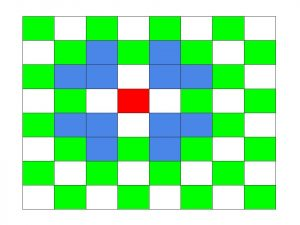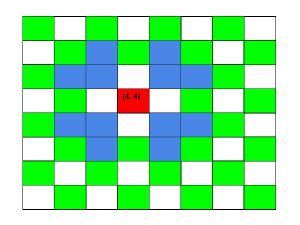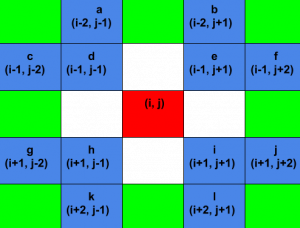# Count all possible position that can be reached by Modified Knight

Given a chessboard of size 8 x 8 and the current position of Mirandote. All the rules of this chess game are same but the knight is modified, we call new knight as “Mirandote”. The moves of Mirandote is given by blue color where its current position is denoted by red color in the following image :The task is to find how many possible positions exist in Chessboard that can be reached by Mirandote in exactly S steps.

Examples:

Input: row = 4, col = 4, steps = 1
Output: 12
All the 12 moves denoted by the following image by blue color :Input: row = 4, col = 4, steps = 2
Output: 55

## Recommended: Please try your approach on {IDE} first, before moving on to the solution.

Solution:

We can observe that all the possible position with respect to current position can be written in the form of row and column. This thing is illustrated by the following image :We can call a function recursively for each possible position and count all the possible position.

Below is the required implementation to find the positions:

 `// C++ implementation to find the ` `// possible positions ` `#include ` `using` `namespace` `std; ` ` `  `// Function to find the positions ` `void` `findSteps(``int` `current_row, ``int` `current_column, ` `               ``int` `curr, ``int` `board_size, ``int` `steps, ` `               ``int``* visited) ` `{ ` `    ``// Bound checking ` `    ``if` `(current_row >= board_size || current_row < 0 ` `        ``|| current_column >= board_size || current_column < 0 ` `        ``|| curr > steps) { ` `        ``return``; ` `    ``} ` ` `  `    ``// If steps is equal to current steps, ` `    ``// that means current position is reached by Mirandote ` `    ``if` `(curr == steps) { ` `        ``*((visited + (current_row)*board_size) + current_column) = 1; ` `        ``return``; ` `    ``} ` ` `  `    ``// Recursive calls for each possible position. ` `    ``// Position of a, b, c, ..., l given in above image. ` `    ``/* a = */` `findSteps(current_row - 2, current_column - 1, ` `                        ``curr + 1, board_size, steps, visited); ` ` `  `    ``/* b = */` `findSteps(current_row - 2, current_column + 1, ` `                        ``curr + 1, board_size, steps, visited); ` ` `  `    ``/* c = */` `findSteps(current_row - 1, current_column - 2, ` `                        ``curr + 1, board_size, steps, visited); ` ` `  `    ``/* d = */` `findSteps(current_row - 1, current_column - 1, ` `                        ``curr + 1, board_size, steps, visited); ` ` `  `    ``/* e = */` `findSteps(current_row - 1, current_column + 1, ` `                        ``curr + 1, board_size, steps, visited); ` ` `  `    ``/* f = */` `findSteps(current_row - 1, current_column + 2, ` `                        ``curr + 1, board_size, steps, visited); ` ` `  `    ``/* g = */` `findSteps(current_row + 1, current_column - 2, ` `                        ``curr + 1, board_size, steps, visited); ` ` `  `    ``/* h = */` `findSteps(current_row + 1, current_column - 1, ` `                        ``curr + 1, board_size, steps, visited); ` ` `  `    ``/* i = */` `findSteps(current_row + 1, current_column + 1, ` `                        ``curr + 1, board_size, steps, visited); ` ` `  `    ``/* j = */` `findSteps(current_row + 1, current_column + 2, ` `                        ``curr + 1, board_size, steps, visited); ` ` `  `    ``/* k = */` `findSteps(current_row + 2, current_column - 1, ` `                        ``curr + 1, board_size, steps, visited); ` ` `  `    ``/* l = */` `findSteps(current_row + 2, current_column + 1, ` `                        ``curr + 1, board_size, steps, visited); ` ` `  `    ``return``; ` `} ` ` `  `int` `countSteps(``int` `current_row, ``int` `current_column, ` `               ``int` `board_size, ``int` `steps) ` `{ ` ` `  `    ``// Visited array ` `    ``int` `visited[board_size][board_size]; ` ` `  `    ``// Initialize visited array to zero ` `    ``for` `(``int` `i = 0; i < board_size; i++) { ` `        ``for` `(``int` `j = 0; j < board_size; j++) { ` `            ``visited[i][j] = 0; ` `        ``} ` `    ``} ` ` `  `    ``int` `answer = 0; ` ` `  `    ``// Function call where initial step count is 0 ` `    ``findSteps(current_row, current_column, 0, ` `              ``board_size, steps, (``int``*)visited); ` ` `  `    ``for` `(``int` `i = 0; i < board_size; i++) { ` `        ``for` `(``int` `j = 0; j < board_size; j++) { ` ` `  `            ``// If value of element is 1, that implies, ` `            ``// the position can be reached by Mirandote. ` `            ``if` `(visited[i][j] == 1) { ` `                ``answer++; ` `            ``} ` `        ``} ` `    ``} ` ` `  `    ``return` `answer; ` `} ` ` `  `// Driver code ` `int` `main() ` `{ ` `    ``int` `board_size = 8, steps = 1; ` `    ``int` `current_row = 4, current_column = 4; ` ` `  `    ``cout << countSteps(current_row, current_column, ` `                       ``board_size, steps); ` `    ``return` `0; ` `} `

Output:

```12
```

Time complexity of above algorithm is O(), where S is the number of steps.

Don’t stop now and take your learning to the next level. Learn all the important concepts of Data Structures and Algorithms with the help of the most trusted course: DSA Self Paced. Become industry ready at a student-friendly price.

My Personal Notes arrow_drop_upCheck out this Author's contributed articles.

If you like GeeksforGeeks and would like to contribute, you can also write an article using contribute.geeksforgeeks.org or mail your article to contribute@geeksforgeeks.org. See your article appearing on the GeeksforGeeks main page and help other Geeks.

Please Improve this article if you find anything incorrect by clicking on the "Improve Article" button below.